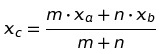# Combined Mean: Definition, Examples

A combined mean is a mean of two or more separate groups, and is found by :

1. Calculating the mean of each group,
2. Combining the results.

## Combined Mean Formula

Watch the video for two worked examples using the combined mean formula:

A combined mean for two sets can be calculated by the formula:

Where:

• xa = the mean of the first set,
• m = the number of items in the first set,
• xb = the mean of the second set,
• n = the number of items in the second set,
• xc the combined mean.

A combined mean is simply a weighted mean, where the weights are the size of each group.

For more than two groups:

1. Add the means of each group—each weighted by the number of individuals or data points,
2. Divide the sum from Step 1 by the sum total of all individuals (or data points).

## Calculating a Combined Mean: Examples

Suppose you are running a survey on math proficiency (as measured by an achievement test) in kindergarten, and you have results from two different schools.

• In school 1, 57 kindergarteners were tested and their mean score was 82.
• In school 2, 23 kindergartners were tested and their mean score was 63.

The combined mean can be calculated by plugging in our numbers into the formula given above:

[(57*82)+(23*63)]/(57+23) = 76.5.

Now suppose you were running a survey on reading speed, as measured by how long it took 1st graders to read a given block of text. Your results come in for five schools:

To calculate the combined mean:

1. Multiply column 2 and column 3 for each row,
2. Add up the results from Step 1,
3. Divide the sum from Step 2 by the sum of column 2.

((189*83) + (46*121) + (89*82) + (50*147) + (12*60)) / (189 + 46 + 89 + 50 + 12) = 94.87
Plug that in your calculator, and the answer you get—94.87—is the combined mean for all five schools; the average reading time for all students.

This same method may be used to combine any number of means.

## References

Beyer, W. H. CRC Standard Mathematical Tables, 31st ed. Boca Raton, FL: CRC Press, pp. 536 and 571, 2002.
Everitt, B. S.; Skrondal, A. (2010), The Cambridge Dictionary of Statistics, Cambridge University Press.
Kotz, S.; et al., eds. (2006), Encyclopedia of Statistical Sciences, Wiley.
Vogt, W.P. (2005). Dictionary of Statistics & Methodology: A Nontechnical Guide for the Social Sciences. SAGE.

CITE THIS AS:
Stephanie Glen. "Combined Mean: Definition, Examples" From StatisticsHowTo.com: Elementary Statistics for the rest of us! https://www.statisticshowto.com/combined-mean/